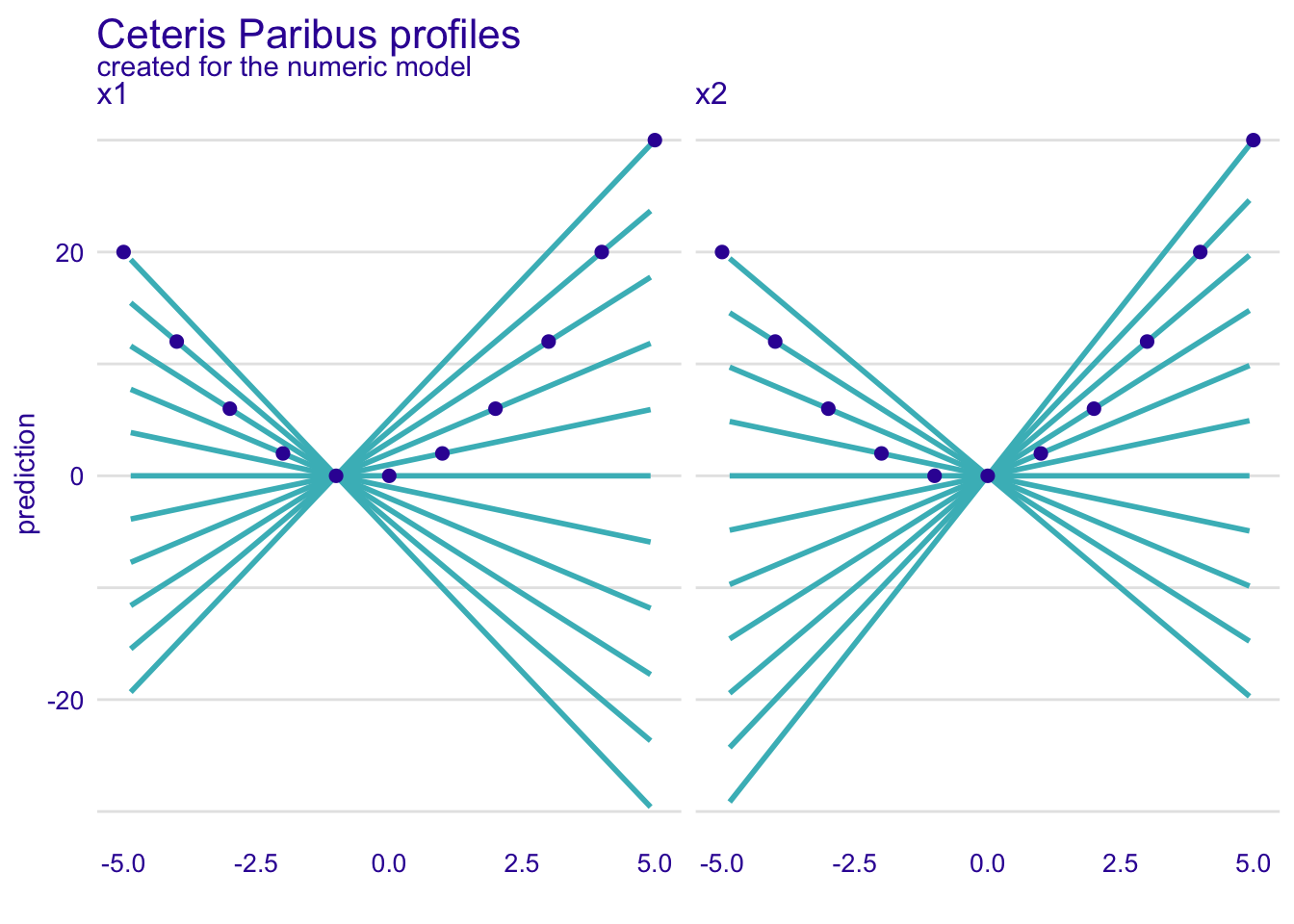# Simulated data

Let’s consider a following problem, the model is defined as

\[ y = x_1 * x_2 + x_2 \]

But \(x_1\) and \(x_2\) are correlated. How XAI methods work for such model?

``````# predict function for the model
the_model_predict <- function(m, x) {
x\$x1 * x\$x2 + x\$x2
}

# correlated variables
N <- 50
set.seed(1)
x1 <- runif(N, -5, 5)
x2 <- x1 + runif(N)/100
df <- data.frame(x1, x2)``````

# Explainer for the models

In fact this model is defined by the predict function `the_model_predict`. So it does not matter what is in the first argument of the `explain` function.

``````library("DALEX")
explain_the_model <- explain(1,
data = df,
predict_function = the_model_predict)``````
``````#> Preparation of a new explainer is initiated
#>   -> model label       :  numeric  (  default  )
#>   -> data              :  50  rows  2  cols
#>   -> target variable   :  not specified! (  WARNING  )
#>   -> predict function  :  the_model_predict
#>   -> predicted values  :  No value for predict function target column. (  default  )
#>   -> model_info        :  package Model of class: numeric package unrecognized , ver. Unknown , task regression (  default  )
#>   -> model_info        :  Model info detected regression task but 'y' is a NULL .  (  WARNING  )
#>   -> model_info        :  By deafult regressions tasks supports only numercical 'y' parameter.
#>   -> model_info        :  Consider changing to numerical vector.
#>   -> model_info        :  Otherwise I will not be able to calculate residuals or loss function.
#>   -> predicted values  :  numerical, min =  -0.1726853 , mean =  7.70239 , max =  29.16158
#>   -> residual function :  difference between y and yhat (  default  )
#>   A new explainer has been created!``````

# Ceteris paribus

Use the `ceteris_paribus()` function to see Ceteris Paribus profiles. Clearly it’s not an additive model, as the effect of \(x_1\) depends on \(x_2\).

``````library("ingredients")
library("ggplot2")

sample_rows <- data.frame(x1 = -5:5,
x2 = -5:5)

cp_model <- ceteris_paribus(explain_the_model, sample_rows)
plot(cp_model) +
show_observations(cp_model) +
ggtitle("Ceteris Paribus profiles")``````# Dependence profiles

Lets try Partial Dependence profiles, Conditional Dependence profiles and Accumulated Local profiles. For the last two we can try different smoothing factors

``````pd_model <- partial_dependence(explain_the_model, variables = c("x1", "x2"))
pd_model\$`_label_` = "PDP"

cd_model <- conditional_dependence(explain_the_model, variables = c("x1", "x2"))
cd_model\$`_label_` = "CDP 0.25"

ad_model <- accumulated_dependence(explain_the_model, variables = c("x1", "x2"))